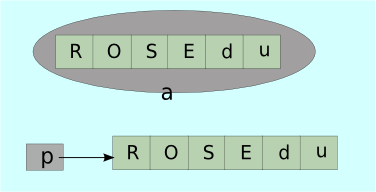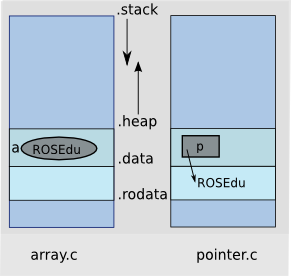# char[] versus char*

Published on August 27, 2011 by
Tagged:

This post will shed some light on the differences between arrays and pointers specifically when it comes about referencing string literals. We will base our discussion on the following two programs:array.c

char a[] = "ROSEdu";
int main(void)
{
a = 'r';
printf("%s\n", a);
return 0;
}

pointer.c

char *p = "ROSEdu";
int main(void)
{
*p = 'r';
printf("%s\n", p);
return 0;
}

Program array.c defines an array of char whose elements are initialized with character string literals, while pointer.c defines a pointer to char and initializes it with the address of a memory area holding a string literal.

Notice array a and pointer p allocations in the image above. Can you make a guess about size of a and size of p?

Next, both programs modify the first character of the string literal "ROSEdu".

Are these two programs equivalent? At the first glance the answer seems to be positive, but let’s have a minute and actually run the code.

$./array rOSEdu$ ./pointer
Segmentation fault

While we could modify array a, our program was killed attempting to modify string literal pointed by p.

We will now have a look at the generated assembly code and notice the section where string literal "ROSEdu" is stored.$gcc -S array.c -o array.s$ cat array.s
.globl a
.data
.type  a, @object
.size  a, 7
a:
.string "ROSEdu"
$gcc -S pointer.c -o pointer.s$ cat pointer.s
globl p
.section        .rodata
.LC0:
.string "ROSEdu"
.data
.type  p, @object
.size  p, 4
p:
.long  .LC0
.text

We can see that array a is stored in data section, which is writable and there is no problem when it is modified. On the other hand, we can notice that p is a pointer stored in data section but it points to a read only memory location, thus accessing it results in Segmentation Fault.

C99 standards (Section 6.7.8) states that:

• contents of the array a is modifiable.
• if an attempt is made to use pointer p to modify the contents of the array, the behaviour is undefined.

So now we see why pointer.c program crashed. gcc decided to store string literal pointed by p into read only data section. One must remark that this is not mandatory, and its implementation dependant.

We invite you to answer following questions:

• What is the sizeof(p) and sizeof(a) in our previous examples?
• What happens if variables a and p are declared on the stack?
• Is it possible for the following expression (const char []){"ROSEdu"} == "ROSEdu" to yield true?